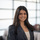# Average Time Interval between a Start and End Time

Looking to get the average time interval between a start and an end time (ex: Given the start and end times for runners in a race, find the average time it takes for a runner to complete the race)? Find exactly how to write this up in each flavor of SQL below!

Redshift

`select avg(extract(epoch from endtime) - extract(epoch from starttime)) as avg_time`

and Postgres

```select
avg(endtime - starttime) as avg_time
```

SQL Server

```select
cast(cast(avg(cast((endtime - starttime) as FLOAT) - floor(cast((endtime - starttime) as FLOAT))) as datetime) as time) as 'Avg Time'```

MySQL

```select
TIME(FROM_UNIXTIME(AVG(UNIX_TIMESTAMP(endtime) - UNIX_TIMESTAMP(starttime)))) AS AVG_TIME```

BigQuery (Standard SQL)

```select
TIME(TIMESTAMP_MILLIS(CAST(avg(DATETIME_DIFF(endtime,starttime,millisecond)) as INT64))) as avg_time```

SQL Server solution inspired by this post